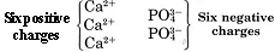# 6.18: Ionic Compounds Containing Polyatomic Ions

$$\newcommand{\vecs}{\overset { \rightharpoonup} {\mathbf{#1}} }$$ $$\newcommand{\vecd}{\overset{-\!-\!\rightharpoonup}{\vphantom{a}\smash {#1}}}$$$$\newcommand{\id}{\mathrm{id}}$$ $$\newcommand{\Span}{\mathrm{span}}$$ $$\newcommand{\kernel}{\mathrm{null}\,}$$ $$\newcommand{\range}{\mathrm{range}\,}$$ $$\newcommand{\RealPart}{\mathrm{Re}}$$ $$\newcommand{\ImaginaryPart}{\mathrm{Im}}$$ $$\newcommand{\Argument}{\mathrm{Arg}}$$ $$\newcommand{\norm}{\| #1 \|}$$ $$\newcommand{\inner}{\langle #1, #2 \rangle}$$ $$\newcommand{\Span}{\mathrm{span}}$$ $$\newcommand{\id}{\mathrm{id}}$$ $$\newcommand{\Span}{\mathrm{span}}$$ $$\newcommand{\kernel}{\mathrm{null}\,}$$ $$\newcommand{\range}{\mathrm{range}\,}$$ $$\newcommand{\RealPart}{\mathrm{Re}}$$ $$\newcommand{\ImaginaryPart}{\mathrm{Im}}$$ $$\newcommand{\Argument}{\mathrm{Arg}}$$ $$\newcommand{\norm}{\| #1 \|}$$ $$\newcommand{\inner}{\langle #1, #2 \rangle}$$ $$\newcommand{\Span}{\mathrm{span}}$$

When polyatomic ions are included, the number of ionic compounds increases significantly. Indeed, most ionic compounds contain polyatomic ions. Well-known examples are sodium hydroxide (NaOH) with OH- as the polyatomic anion, calcium carbonate (CaCO3), and ammonium nitrate (NH4NO3), which contains two polyatomic ions: NH+ and NO3-.

A list of the more important polyatomic ions is given in the following table, which can be used for reference while learning the charges of polyatomic ions. A great many of them are oxyanions (polyatomic ions that contain oxygen).

Table $$\PageIndex{1}$$ Ions

 Charge Name Formula -3 Phosphate PO43- Arsenate AsO43- -2 Carbonate CO32- Peroxide O22- Sulfate SO42- Sulfite SO32- Chromate CrO42- Dichromate Cr2O72- Hydrogen phosphate HPO42- -1 Hydrogen carbonate (bicarbonate) HCO3- Superoxide O2- Hydrogen sulfate HSO4- Dihydrogen phosphate H2PO4- Hydroxide OH- Nitrate NO3- Nitrite NO2- Acetate C2H3O2- or CH3COO- Cyanide CN- Permanganate MnO4- Perchlorate ClO4- Chlorate ClO3- Chlorite ClO2- Hypochlorite ClO- +1 Ammonium NH4+ Hydronium H3O+

The properties of compounds containing polyatomic ions are very similar to those of binary ionic compounds. The ions are arranged in a regular lattice and held together by coulombic forces of attraction. The resulting crystalline solids usually have high melting points (1500 °F for CaCO3) and all conduct electricity when molten.

Most are soluble in water and form conducting solutions in which the ions can move around as independent entities. In general polyatomic ions are colorless, unless, like CrO42– or MnO4, they contain a transition-metal atom. The more negatively charged polyatomic ions, like their monatomic counterparts, show a distinct tendency to react with water, producing hydroxide ions; for example,

$\ce{PO_{4}^{3-} + H_{2}O \rightarrow HPO_{4}^{2-} + OH^{-}}$

It is important to realize that compounds containing polyatomic ions must be electrically neutral. In a crystal of calcium sulfate, for instance, there must be equal numbers of Ca2+ and SO42– ions in order for the charges to balance. The formula is thus CaSO4. In the case of sodium sulfate, by contrast, the Na+ ion has only a single charge. In this case we need two Na+ ions for each SO42–ion in order to achieve electroneutrality. The formula is thus Na2SO4.

Structurally, polyatomic ions are similar to the ionic solids we saw earlier. An example of a simple ionic compound, NaCl, is seen below, alongside a more complex ionic solid, AgClO3-. Notice how both are tightly packed and form a repeating pattern, which lends both compounds strength and brittleness. In the Silver Chlorate (AgClO3-), however, polyatomic ions are present where the Cl- ions are present in the Sodium Chloride (NaCl).On the left is the lattice structure of the ionic solid NaCl, with Na represented by the green spheres and Cl represented by the purple spheres. On the right is the lattice structure of AgClO3, with the silver spheres representing Ag and the red and green molecules representing the polyatomic ion ClO3

Example $$\PageIndex{1}$$ : Ionic Formula

What is the formula of the ionic compound calcium phosphate?

Solution It is necessary to have the correct ratio of calcium ions, Ca2+, and phosphate ions, PO43–, in order to achieve electroneutrality. The required ratio is the inverse of the ratio of the charges on ions. Since the charges are in the ratio of 2:3, the numbers must be in the ratio of 3:2. In other words the solid salt must contain three calcium ions for every two phosphate ions:The formula for calcium phosphate is thus Ca3(PO4)2.